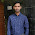## Electrical Machine Competitive Questions with Answers:

 A field excitation of 20A in a certain alternator results in an armature current of 400A in short circuit and a terminal voltage of 2000V on open circuit. The magnitude of the internal voltage drop within the machine at a load current of 200A is [GATE2009]
(a) 1V
(b) 10V
(c) 100V
(d) 1000V

 Figure shows the extended view of a 2 pole dc machine with 10 armature conductors.Normal brush positions are shown by A and B, placed at the interpolar axis. If the brushes are now shifted, in the direction of rotation, to A' and B' as shown, the voltage waveform VA'B' will resemble. [GATE]

A 220V,50Hz,single-phase induction motor has the following connection diagram and winding orientations shown.MM' is the axis of the main stator winding(M1M2) and AA' is that of the auxiliary winding (A1A2). Directions of the winding axes indicate direction of flux when currents in the windings are in the directions shown. Parameters of each winding are indicated. When switch S is closed, the motor [GATE]

(a) Rotates clockwise
(b) Rotates anticlockwise
(c) Does not rotate
(d) Rotates momentarily and comes to a halt

 The electromagnetic torque Te of a drive, and its connected load torque Tl are as shown below. Out of the operating points A,B, C and D, the stable ones are [GATE 2007]

 In a transformer, zero voltage regulation at full load is [GATE 2007]
(a) Not possible
(b) Possible at unity Power factor load
(c) Possible at leading Power factor load
(d) Possible at lagging Power factor load

 The DC motor, which can provide zero speed regulation at full load without any controller is [GATE 2007]
(a) Series
(b) Shunt
(c) Cumulative Compound
(d) Differential Compound

 A single phase 10kVA, 50 Hz transformer with 1kV primary winding draws 0.5A and 55W, at rated voltage and frequency, on no load. A second transformer has a core with all its linear 2 times the corresponding dimensions of the first transformer. The core material and lamination thickness are the same in both transformers. The primary windings of both the transformers have the same number of turns. If a rated voltage of 2kV at 50Hz  is applied to the primary of the second transformer, then the no load current and power, respectively, are  [GATE2012]
(a) 0.7 A,77.8W
(b) 0.7A,155.6 W
(c) 1A,110W
(d) 1A,220W

 The locked rotor current in a 3-phase, star connected 15kW, 4-pole, 230V, 50Hz induction motor at rated conditions is 50A. Neglecting losses and magnetizing current, the approximate locked rotor line current drawn when the motor is connected to a 236V, 57Hz supply is
[GATE2012]
(a) 58.5A
(b) 45.0A
(c) 45.7A
(d) 55.6A

 A 220V, 15kW,1000rpm shunt motor with armature resistance of 0.25Ω, has a rated line current of 68A and a rated field current of 2.2A. The change in field flux required to obtain a speed of 1600 rpm while drawing a line current of 52.8A and a field current of 1.8A is
[GATE2012]
(a) 18.18% increase
(b) 18.18% decrease
(c) 36.36% increase
(d) 36.36% decrease

 In 8 - pole wave connected motor armature, the number of parallel paths are
(a) 8
(b) 4
(c) 2
(d) 1

9:13 AM

#### 1 comment:

1.Question No 6 the answer is differential compound Because as the load increases the resistance drop increase and flux decreases.If drop in speed due to armature resistance is equal to rise in speed due to reduction in flux then the speed regulation will be zero